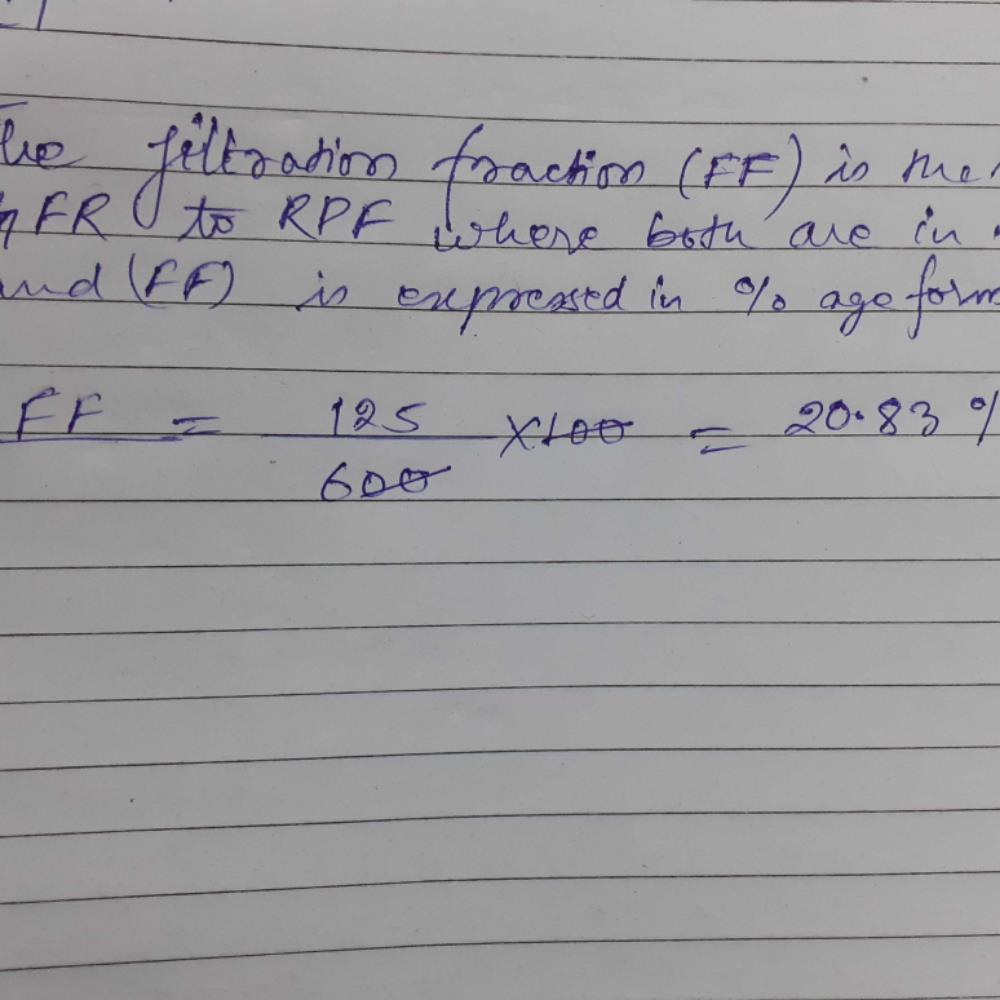Courses

# The filtration fraction is the ratio of GFR to RPF where both the values are in ml/min and FF is expressed in percentage. Calculate FF for a normal adult human being, if RPF= 600ml/min :a)2.08%b)20.73%c)10.38%d)20.83%Correct answer is option 'D'. Can you explain this answer? Related Test: Test: Excretory Products And Their Elimination- 1

## NEET Question

By Ayaana Dastur · Aug 10, 2019 ·NEET
Rahul Kumar answered Aug 10, 2019Wafa Majid Qazi answered Aug 07, 2020
FF = Filtration fraction,
RPF = Renal plasma flow,
GFR = Glomerular filtration rate = 125ml (for healthy human),

FF = GFR/RPF,
So, FF% = GFR/RPF ×100
= 125/600 ×100
= 125/6
=20.83%

Jasmail Kaur answered Mar 03, 2020
FF = (125/600)*100 = 20.83%